## Introduction

Quantum metrology exploits particle entanglement in the probe state to enhance the precision of parameter estimation beyond what is reachable with classical resources (see refs 1,2 for reviews). The role of particle entanglement in the estimation of a single parameter has been clarified3,4,5,6 and investigated experimentally in Mach-Zehnder interferometers (MZIs)7. However, much less is known about the role of particle entanglement in the joint estimation of multiple parameters. Multiparameter estimation is relevant in many practical applications, including quantum imaging8, quantum process tomography9, as well as probing of biological samples10. Interestingly, the theory of multiphase estimation does not follow trivially from what is known about the single parameter case11,12. Indeed, ultimate multiphase estimation bounds are not saturable in general13, due to the non-commutativity of the operators generating the phase shift transformations14,15. First insights on this scenario have been recently reported16,17,18,19,20,21,22.

A natural platform for multiparameter quantum metrology is provided by multiport interferometry, generalizing conventional two-mode interferometry. Recent progresses in the realization of multiport devices have been achieved by exploiting integrated photonics23,24,25,26,27,28,29,30,31. Three- and four-port beam-splitters (tritters and quarters) have been produced with integrated optics31,32,33,34. This paves the way toward the realization of multiarm interferometers created by two tritters (quarters) in succession35. Quantum-enhanced single parameter estimation in integrated interferometers has been theoretically predicted17, while multiparameter estimation in multi-arm interferometers has been examined and compared with the sensitivity achievable by multiple single-parameter estimation18.

In this manuscript we provide conditions of useful particle entanglement for the simultaneous estimation of multiple phases. We study a general multimode scenario where each particle is treated as a qudit. Furthermore, we adapt the theory to the case of multiarm Mach Zehnder interferometers (MMZIs) considering an experimentally relevant framework, with multiphoton Fock states as probe and photon counting measurement. Our analysis generalizes the case of twin-Fock MZI which has attracted large experimental7,36,37,38 and theoretical39,40,41 interest for quantum-enhanced single phase estimation. From the analysis of the Fisher information and employing an adaptive multiphase estimation, we predict a multiparameter estimation sensitivity beyond the limit achievable with separable qudit probe states.

## Results

### Multiparameter estimation

We consider here the estimation of a n-dimensional vector parameter λ = (λ1,, λn)11,12. In our benchmark, every parameter corresponds to a phase to be estimated in a multiarm interferometer. A general approach (see Fig. 1a) consists in preparing a probe state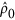, applying a λ -dependent unitary transformation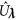and performing independent measurements on ν identical copies of the output state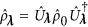. The measurement is described by a positive-operator valued measure (POVM), i.e. a set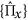of positive operators satisfying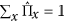,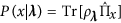being the probability of the detection event x. Finally, the sequence x ≡ (x1,, xν) of ν measurement results is mapped into a vector parameter Λ( x ) = (Λ1( x ),, Λn( x )), representing our estimate of λ . A figure of merit of multiparameter estimation is the covariance matrixwhere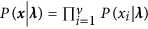and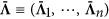is the mean value of the estimator vector. For locally unbiased estimators (i.e.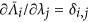) the covariance matrix is bounded, via the Cramer-Rao theorem11, as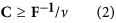(in the sense of matrix inequality), whereis the Fisher information matrix (FIM). Notice that Eq. (2) can be derived only when the FIM is invertible. The equality sign in Eq. (2) is saturated asymptotically in ν by the maximum likelihood estimator11. Here we quantify the phase sensitivity by the variance of each estimator, (δλj)2 ≡ Cj,j. We have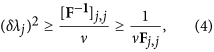where the first inequality is due to (2) and the second follows from a Cauchy-Schwarz inequality (see Supplementary Information). Since 1/(ν Fj,j) is the Cramer-Rao bound for single parameter estimation, inequality (4) tells us that sensitivity in the estimation of λj can be optimized when fixing all the other parameters to known values. We will also consider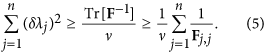The right-hand side inequality in Eqs (4) and (5) is saturated if and only if the FIM is diagonal. Furthermore, the FIM is bounded by the quantum Fisher information matrix (QFIM): F ≤ FQ (in the sense of matrix inequality), where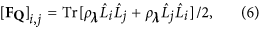and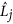is the symmetric logarithmic derivative of ρλ with respect to parameter λj, defined by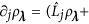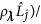2 10. In the single parameter case, the QFIM reduces to a single scalar quantity and it is always possible to find a POVM for which F = FQ and δλ = 1/FQ holds42,43. In contrast, in the multiparameter case, it is generally not possible to achieve the Cramer-Rao bound13,14,15.

### Sensitivity bounds for qudit-separable states

In the following we consider the estimation of n parameters in a system made of d = n + 1 modes (e.g. the number of arms in a MMZI, see below). A single particle occupying the n + 1 modes is generally indicated as a qudit. The notion of qudit generalizes the concept of qubit (a two-mode particle, n = 1) and is relevant in multimode interferometry2. Here we set sensitivity bounds for multiparameter estimation when the probe state is qudit-separable. A stateof N qudits is said to be qudit-separable if it can be written as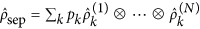, where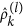(l = 1,, N) is a single qudit state, pk > 0 and ∑kpk = 1. A state which is not qudit-separable is qudit-entangled. We take the generator of each phase shift,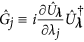(j = 1, …, n labels the parameter), to be local in the qudit, i.e. it can be written as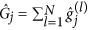where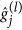is an arbitrary operator acting on the lth qudit. In particular, the transformationdoes not create entanglement among the N qudits. For simplicity, we will take the same operator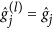for each particle. For a generic separable probe state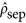, the inequality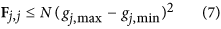holds for all possible POVMs (see Supplementary Information), where gj,max and gj,min are the maximum and minimum eigenvalue of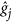, respectively. Inequality (7) gives a bound on the diagonal elements of the FIM. It corresponds, via the inequality (δλj)2 ≥ 1/ν Fj,j, to a bound on the sensitivity reachable with qudit-separable states for the estimation of the single parameter λj, when all other parameters are set to zero. Inequality (7) can be always saturated by optimal states and measurements (see Supplementary Information). For the estimation of a single parameter, the violation of Eq. (7) is a necessary and sufficient condition of useful qudit entanglement2,4: only those qudit-entangled states that violate Eq. (7) allow to estimate the parameter λj with a sensitivity overcoming the one reachable with any qudit-separable state. Regarding the simultaneous estimation of multiple parameters, we can use Eq. (7) and the chain of inequalities (4) to obtain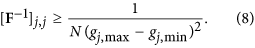Inequality (8) is a bound of sensitivity in the estimation of the single parameter λj with qudit-separable states, when all the parameters are unknown. Summing Eq. (8) over all parameters, we obtain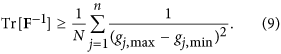According to Eqs (8) and (9), for qudit-separable states such that the FIM is invertible, we recover – at best – the shot noise scaling of phase sensitivity, δλjN−1/2, which also characterizes single parameter estimation3,4. Notice that the quantity (gj,max − gj,min)2 is equal to one for any qubit transformation and might be larger than one for general qudit transformations. We finally recall that the phase estimation scenario we are considering – as well as the notion of useful qudit-entangement – refers to interferometric scheme involving liner qudit transformations and multiple independent measurements done with identical copies of the same probe. Inequalities (8) and (9) have no concern with the qudit-entanglement of the initial probe state for (nonlinear) parameter dependent processes that entangle/disentangle the probe or non-independent multiple measurements.

### Multimode Mach-Zehnder interferometry

In the following we discuss the estimation of a phase vector ϕ = (ϕ1, …, ϕn) in a MMZI (see Fig. 1b,c). The MMZI can be obtained by cascading a d-mode balanced beam-splitter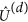, a phase shift transformation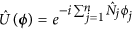, being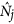the photon-number operator for the ith mode, and a second multiport beam-splitter. The d-mode beam-splitteris the natural extension of the standard 50-50 beam-splitter to more than two optical input-output modes41. Hence, the MMZI can be adopted as a benchmark to investigate simultaneous estimation of n = d − 1 optical phases. Indeed, it allows for a direct comparison between classical and quantum probe states and represents a flexible platform for the analysis of multiparameter scenario by changing the unitary transformation of the input and output multiport beam-splitters.

In order to adapt the discussion of the previous section, we consider N particles as input of the MMZI and identify a single particle in the d arms of the interferometer as a qudit, whose Hilbert space has thus dimension d. The generator of phase shift in the jth mode is. One can thus writewhereas the operator projecting the lth qudit on the jth mode. Finally, gj,max − gj,min = 1 and the inequalities (8) and (9) read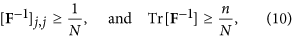respectively. The violation of one of these inequalities in the MMZI is a signature of useful qudit-entanglement in the probe state.

The recent experimental implementation of symmetric multiport beam-splitting31,32,33,34, by adopting integrated platforms, paves the way toward the future realization of optical MMZIs. For d = 3 modes, the tritter matrix, corresponding to its unitary transformation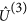, has diagonal elementsand off-diagonal elements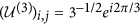with i ≠ j. For d = 4 modes, the quarter matrix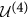is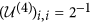and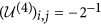for i ≠ j. The overall matrix for the MMZI is then obtained as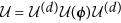. The phase vector is estimated from the measurement of the number of particles in each mode. As probe, we focus on multimode Fock states with a single photon in each input mode of the interferometer18, |1, 1, 1〉 and |1, 1, 1, 1〉 for the three- and four-mode MZI, respectively. Here, |1, 1, 1〉 ≡ |1〉1 |1〉2 |1〉3 (and analogous definition for |1, 1, 1, 1〉), where |1〉j is a Fock state identifying a single particle in the jth mode.

For the three-mode MZI, the results of the calculation for F−1 are shown in Fig. 2a–c. Analytic expression of the FIM is reported in the Supplementary Information. We observe that Tr[F1] and the diagonal elements [F1]1,1 and [F1]2,2 depend on the phases ϕ1 and ϕ2. Notably, the inequalities (10) are violated at certain optimal values of the parameters, signaling that the Fock state |1, 1, 1〉 contains useful qudit entanglement: we find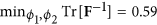(see Fig. 2a) and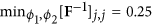(see Fig. 2b,c), which are smaller than the bound for qudit-separable states Tr[F−1] = 0.667 and [F−1]j,j = 0.33 (here N = 3 and n = 2), respectively. Additionally, we observe characteristic features. (i) FFQ, in particular, the minimum value of Tr[F−1] is greater than the corresponding minimum value of the QFIM: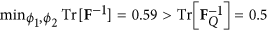(see Fig. 2a). (ii) The FIM is not always invertible: at the phase values for which det F = 0 the bound (2) is not defined. Around these points (white regions in Fig. 2a–c) [F1]1,1 and/or [F1]2,2 diverge. (iii) The working points to obtain the minimum of the multiparameter bound do not lead to symmetric errors on the single parameters ϕ1 and ϕ2. More specifically, when Tr[F−1] = 0.59, the bounds for the error on the single parameters are different: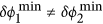. This is obtained for instance for working point Q1 = (ϕ1,ϕ2) = (0.892, 2.190), leading to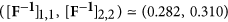and for working point Q2 = (ϕ1,ϕ2) = (2.190, 0.892), leading to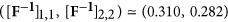, see Fig. 2a. In summary, with this choice of probe state and measurement it is not possible to saturate the quantum Cramer-Rao inequality simultaneously for the two parameters. Furthermore, according to point (iii) an adaptive estimation strategy (which we discuss below) is necessary to obtain the minimum sensitivity on both parameters with symmetric errors, and thus saturate the multiparameter Cramer-Rao bound.

We have repeated the above analysis for the four-mode interferometer (d = 4) with two unknown phases, ϕ1 and ϕ2, and a known control phase ϕ0 (see Fig. 1c). This configuration allows a comparison between three- and four-arm interferometers for the two parameter estimation. In the latter case the control phase ϕ0 gives us an additional degree of freedom. We choose as input the Fock State |1, 1, 1, 1〉. In Fig. 2d–f the results of our calculations are reported for a fixed value of ϕ0, as well as the numerical analysis of det F. We observe that as in the previous cases the FIM depends on the value of the parameter to be estimated. Furthermore, also in the four-mode the achievable sensitivity falls below the bound (10) for separable states: we have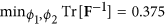,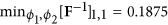and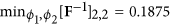which are below the bounds 0.5 and 0.25 given by Eq. (10) (N = 4 and n = 2, here), respectively. The most notable difference with respect to the previous case is that the QCRB is achieved, for instance in working point O1 = [π, π]. In addition, both diagonal terms are equivalent and only a two step adaptive protocol is needed to reach the QCRB for any arbitrary phase vector (see discussion below).

We have also compared the obtained results with the one achievable with other probe states. For instance, we consider a set of distinguishable particles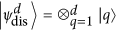(where |q〉 stands for a single photon on mode kq), or an input coherent state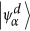on input mode k1 with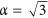for d = 3 (α = 2 for d = 4) and no phase reference. We obtain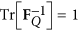for both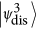and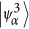, within the bound Tr[F−1] ≥ 0.667 given by Eq. (10) for separable inputs. Similarly,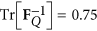for both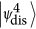and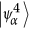, within the bound Tr[F−1] ≥ 0.5. Results are summarized in Tables A and B.

In this section we present the adaptive estimation protocols required to maximize the precision on the simultaneous estimation of two arbitrary phases in a three- and four- mode MZI. The resources (the number of independent measurements ν) are split between multiple steps. A first step is needed to obtain a rough estimate of the unknown phases and requires a small subset of the resources which becomes negligible when the number of repetitions ν of the experiment is large enough. The subsequent steps exploit the available information to optimize the estimation procedure.

Regarding the three-mode interferometer, the above analysis has identified working points (Q1 and Q2) where the minimum uncertainty for the estimation of the two phases ϕ1 and ϕ2 does not give the same error on the two individual parameters. To overcome this limitation – and obtain approximatively a symmetric error in the joint estimation of the two phases – we exploited a three-step adaptive algorithm. The protocol requires ν independent measurements and the adoption of controlled phase shifts ψi on modes ki, with i = 1, 2, which have to be tuned during the protocol to perform the estimation at different working points (see in Fig. 1b). In a first step, we set ψ1,2 = 0 and obtain a rough estimate of the phases ϕi after a number of measurements much smaller than ν. Then, in step 2 the tunable phases ψi are adjusted so that ϕi + ψi on arms 1 and 2 are set to be close to the working point Q1. In this step essentially half of the remaining resources are spent so as to obtain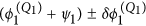and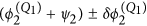with an adequate estimator. Here,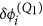represent respectively the estimation and the uncertainty of ϕi around working point Q1. In step 3 the same procedure is repeated for working point Q2. Finally the tunable phases ψ1.2 are subtracted so to recover ϕ1,2 ± δϕ1,2. The results of the adaptive algorithm are shown in Fig. 3a–d. Half of the measurements (ν1 = ν/2) are performed at point Q1, where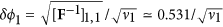and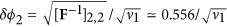, while the other half (ν2 = ν/2) are performed at point Q2, whereand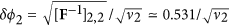. The expected error on a single phase δϕi after the two steps is then obtained as an appropriate combination of the values on the points Qi. More specifically, as the Fisher information is additive, the overall FIM reads F = ν1F1 + ν2F2, where Fi is the FIM in working points Qi. We observe that the protocol permits to achieve the bound of the working point, which for ν1 = ν2 is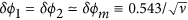. Note that the bound is lower than the bound (10) for separable states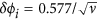.Figure 3: Numerical simulation of adaptive estimation of two phases, ϕ1 and ϕ2 with the three-mode interferometer injected by a |1, 1, 1〉.

The adaptive scheme for the four-mode interferometer is slightly different: in this case there are optimal working points, as the point O1, see Fig. 2, where QCRB is achieved for both phases. To reach the QCRB for arbitrary phases, we thus apply a two-step adaptive protocol. In the first step, we obtain a rough estimate of the parameters with an initial error δ. Then, in the second step we apply two supplementary phases ψ1 and ψ2 to translate the working point of the protocol to the neighbourhood of O1. It should be noticed that a convergent estimation protocol in the second step requires to set ϕ0 such that the quantity Tr[F−1] has no singularities. Note that the more ϕ0 deviates from ϕ0 = 0, the larger is the regular region around O1 (see Supplementary Information). The price to pay is a slightly increasing the error in the estimation process. The value of ϕ0 has to be chosen in order to move the singularity away from a neighbourhood of O1 larger than the inital error δ of the first step. The results of the protocol for the four-mode case with ϕ0 = 0.01 are then shown in Fig. 4a,b. Similarly to the three-mode case, we observe that the protocol permits to achieve the bound of the working point, which is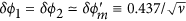for ϕ0 = 0.01 (plane in Fig. 4), while the quantum Cramer-Rao bound reads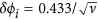. This shows that achieving a convergent numerical protocol leads to a slight decrease in phase sensitivity due to singular points in the neighborhood of the working regions. Also in this case, the adaptive protocol allows to reach a sensitivity overcoming the bound of separable state for any vector parameter.Figure 4: Numerical simulation of adaptive estimation of two phases, ϕ1 and ϕ2 with the four-mode interferometer injected by a |1, 1, 1, 1〉, for ϕ0 = 0.01 and ν = 10000 independent measurements.

## Conclusions

In this manuscript we have developed the general theory of quantum-enhanced multiphase estimation. In particular, we provide conditions of useful qudit-entanglement for the simultaneous estimation of multiple phases below the ultimate sensitivity limit achievable with qudit-separable states. We have focused on interferometers involving linear qudit transformations and multiple independent measurements. In a realistic experimental scenario, using multi-mode Mach-Zehnder interferometers and photo-counting measurements, Fock state probes can be exploited for multiphase estimation with quantum-enhancement phase sensitivity. With respect to the estimation of a single phase, where Fock states are known to be a useful resource, our analysis evidences a rich scenario: most notably, the phase sensitivity strongly depends on the phase value (the Cramer-Rao bound being not always definite) and on the interferometer configurations such as the three- and four-mode interferometers. Finally, we discuss and numerically simulate an adaptive estimation protocol which permits to achieve the expected bounds for any vector parameter. The adaptive strategy becomes crucial in multiparameter estimation since the simultaneous saturation of the ultimate limits for all parameters is in general not guaranteed.

During the completion of this manuscript, a first implementation of a tritter-based interferometer for single-phase estimation has been reported45.